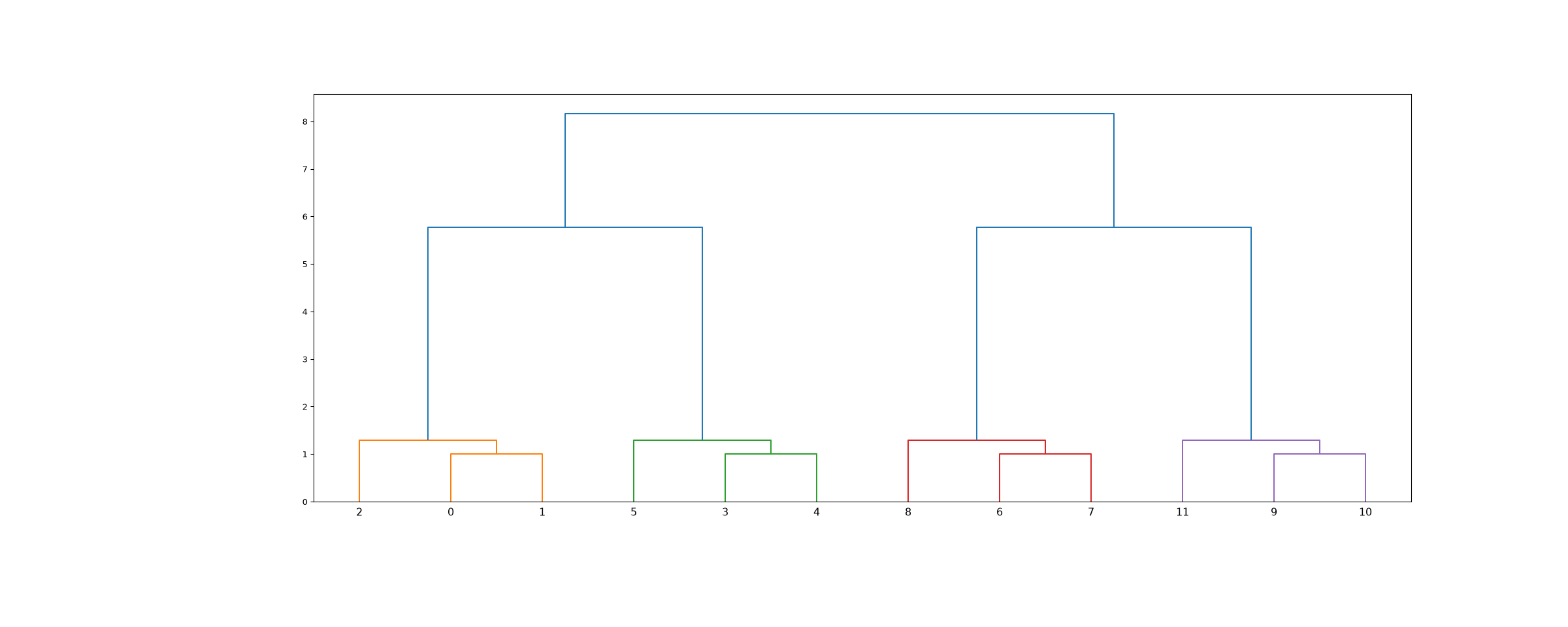# scipy.cluster.hierarchy.leaves_list¶

scipy.cluster.hierarchy.leaves_list(Z)[source]

Return a list of leaf node ids.

The return corresponds to the observation vector index as it appears in the tree from left to right. Z is a linkage matrix.

Parameters
Zndarray

The hierarchical clustering encoded as a matrix. Z is a linkage matrix. See `linkage` for more information.

Returns
leaves_listndarray

The list of leaf node ids.

`dendrogram`

Examples

```>>> from scipy.cluster.hierarchy import ward, dendrogram, leaves_list
>>> from scipy.spatial.distance import pdist
>>> from matplotlib import pyplot as plt
```
```>>> X = [[0, 0], [0, 1], [1, 0],
...      [0, 4], [0, 3], [1, 4],
...      [4, 0], [3, 0], [4, 1],
...      [4, 4], [3, 4], [4, 3]]
```
```>>> Z = ward(pdist(X))
```

The linkage matrix `Z` represents a dendrogram, that is, a tree that encodes the structure of the clustering performed. `scipy.cluster.hierarchy.leaves_list` shows the mapping between indices in the `X` dataset and leaves in the dendrogram:

```>>> leaves_list(Z)
array([ 2,  0,  1,  5,  3,  4,  8,  6,  7, 11,  9, 10], dtype=int32)
```
```>>> fig = plt.figure(figsize=(25, 10))
>>> dn = dendrogram(Z)
>>> plt.show()
```